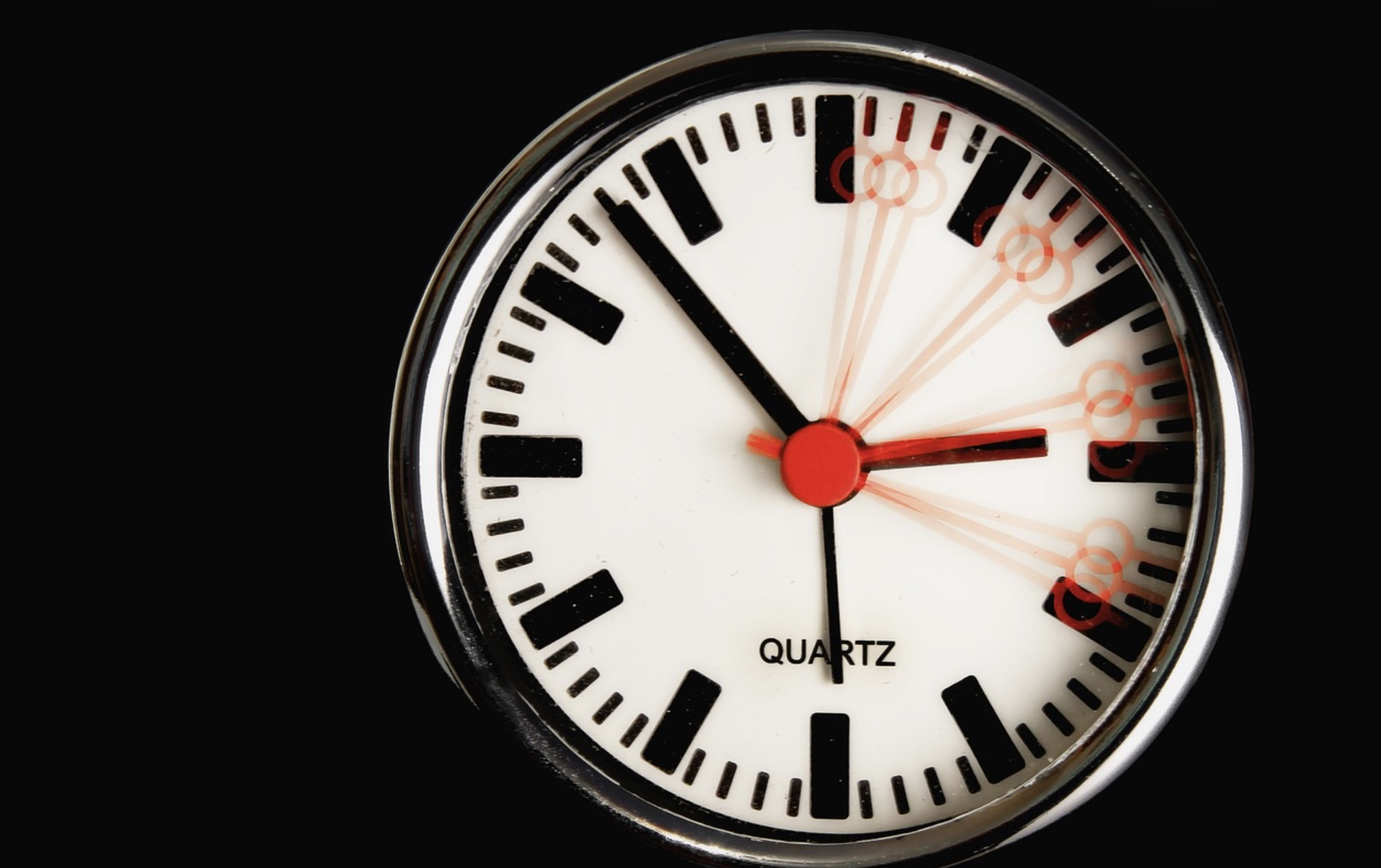France +33 77 485 85 info@armen-instrument.com# How to measure movement, torque or forceThe measurement of force, movement (acceleration), and torque are connected to the same piezoelectric that measures dynamic and static pressures.An accelerometer measures acceleration by sensing the movement of the surface it is attached. The device produces an electric output signal that shows the rate of acceleration. The measured mass is what yields the force.

Torque, on the other hand, is the twisting force experienced on pulleys, shafts, bars, and any other devices that rotate. This torque is calculated by multiplying the force product by the radius of the area on which the force acts. The unit of torque is pound-feet or Newton meter.

## Using a Piezoelectric Sensor

The piezoelectric force sensor is the most common acceleration and dynamic sensor. When squeezed, the piezoelectric sensor creates a voltage – the word piezo means “to squeeze” in Greek. The high impedance signal from the piezoelectric crystal goes through an amplifier, where it is converted into a low impedance electric signal to make it appropriate for a digital storage oscilloscope. It is the digital storage that analyzes the signal before decay.

## Ways to Measure Dynamic Force

The way you measure dynamic force depends on the application. You can measure it as tensile, compression or torque force. Common applications are sliding or spring friction forces, clutch release forces, chain tensions, and peel strengths of labels, pull tabs, and laminates.

A piezoelectric sensor, which uses load cells to generate electric signals, is as rigid as a piece of solid steel. Thanks to their strength, the devices are mounted as part of the machine. The rigidity also gives them high natural frequency. That makes the devices appropriate for measuring quick transient forces such as those generated from a metal to metal impact. For the measurements to be accurate, the measuring devices need to have a higher frequency than the device to be measured. Whenever the frequency of the measured frequency nears the sensing device’s frequency, there are chances that an error might occur.

## Using an Impact Flowmeter

An impact flowmeter senses force. However, the flowmeter only measures a free-flowing bulk solid flow rate. The chute directs the flow of the material to the sensing plates. It is the impact force on the plate that determines the flow rate.

The sensing plate only moves in the horizontal plane – the impact force, therefore, gives the plate a horizontal deflection, and that is how force is measured. Sensing devices use an LVDT (linear variable differential transformer). The device then converts the voltage from the LVDT into pulse frequency modulated signal, which is transmitted to the control system.

An impact flowmeter can be used as an alternative to a weighing system for measurements and control of the flow of bulk solids.

## Acceleration and Vibrations

Modern accelerometers are more advanced in design and they cost less than other early devices. Early devices were analog but later converted into digital microprocessors. The airbag controls in cars apply the hybrid micro-electromechanical systems, also known as MEMS. Modern MEMS are used in airbag controls, impact detection, and in anti-lock braking systems. The accelerometers are available for different frequencies from 0.1 to 1,500 Hz.